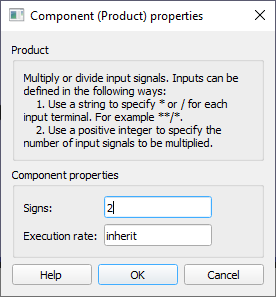# Product

Description of the Product component in Schematic Editor, which multiplies values of its input values.

## Description

The Product component multiplies values of the input values.

If the signal is connected to the “÷” terminal, its value will be converted to reciprocal value (1/in). If the signal is connected to the “x” terminal, its value will remain unchanged.

The number of inputs of the sum component is defined using Signs property. If it is defined by integer N, then the block will have N inputs, and all inputs will be “x”, as shown in Figure 2.

If some of the values are intended for division, then a string containing characters “*” and “/” must be set on Signs property as illustrated in Figure 3.

Product component can have one or more inputs which can be scalar or vectors. The output is determined using the following rules:
• If there is one terminal and scalar value on input, the input value will be passed to the output (Example 1)
• If there is one terminal and vector value on input, the vector product will be performed. The output will be scalar (Example 2)
• If there are multiple terminals and all inputs are scalar, product value will be determined by multiplying all the inputs. The output will be scalar. (Example 3)
• If there are multiple terminals and all inputs are vectors, the output will be vector and output value is determined by multiplying values element-wise . All input vectors must be the same length (Example 4)
• If some inputs are scalars and some vectors, the output will be vector and value will be determined by multiplying inputs element-wise (if vector) and using scalar values (if scalar). (Example 5)
 in 1 in 2 out Example 1 2(connected to ÷) - 1/2 = 0.5 Example 2 [1, 2, 1/2, 1/3, 5] - 1 * 2 * 1/2 *1/3 * 5 = 1.666 Example 3 2 3 6 Example 4 [3, 3, 1/2] [3, 1/2, 4] out = 3 * 3out = 3 * 1/2out = 1/2 * 4 out = [9, 1.5, 2] Example 5 4 [1, -3, 1/2] out = 4 * 1out = 4 * (-3)out = 4 * 1/2 out = [4, -12, 2]

## Ports

• Input (in)
• Input signals to be multiplied. Care must be taken when connecting values 0 to “÷” terminal. In that case division by zero will occur and the result will be unpredictable.
• Supported types: uint, int and real.
• Vector support: yes.
• Output (out)
• Result of operation performed by the product component.
• Supported types: uint, int and real.
• The output type is inherited from the input signal.
• Vector support: yes.
• The vector length is calculated based on the number of inputs and input size. If the number of inputs is 1, the output will be scalar. If the number of inputs is larger than 1, the output will be scalar if all inputs are scalar, and vector if any input is vector.

## Properties• Signs
• Type in a combination of characters that will define the number of input terminals. If the value is positive integer N, N “x” terminals will be created. If the value is a string containing “*” and “/“ characters, the number of terminals will be the same as the number of characters.
• Execution rate
• Type in the desired signal processing execution rate. This value must be compatible with other signal processing components of the same circuit: the value must be a multiple of the fastest execution rate in the circuit. There can be up to four different execution rates, but they must all be multiple of the basic simulation timestep. To specify the execution rate, you can use either decimal (e.g. 0.001) or exponential values (e.g. 1e-3) in seconds. Alternatively, you can type in ‘inherit’ in which case the component will be assigned execution rate based on the execution rate of the components it is receiving input from.